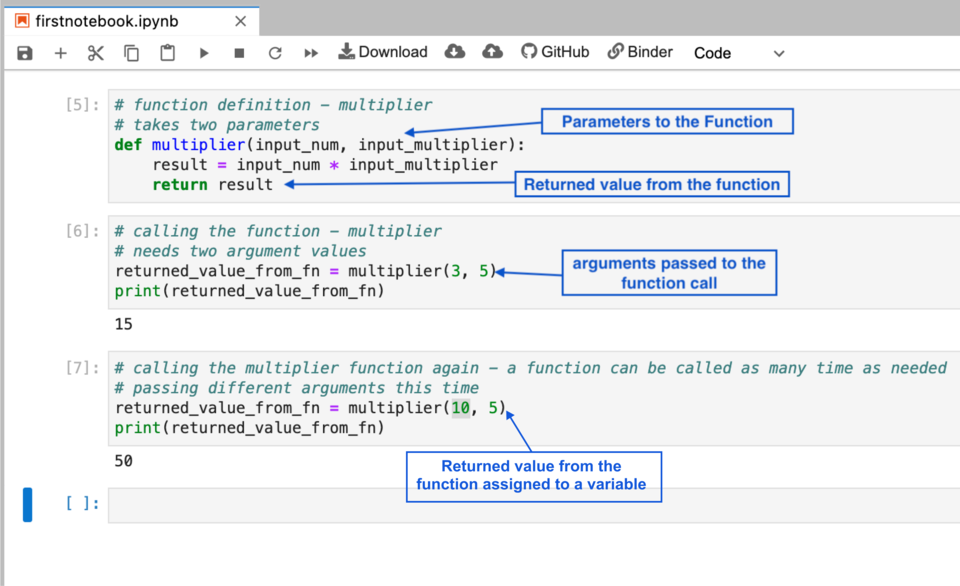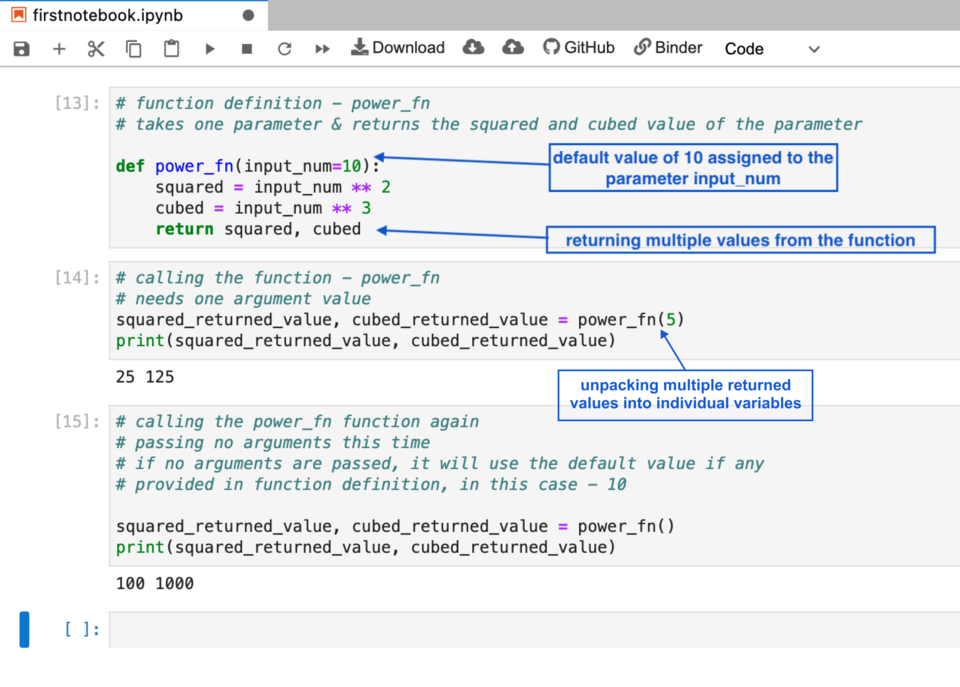{body}View your saved Course or Program Packages containing pricing and detailed curriculum.Have any questions? We'll call you.

Fill out the form below and a Learning Advisor will reach out at a time convenient for you.

Please pick a valid date and time between 9 AM and 8 PM eastern (Monday to Friday)

By clicking "Book a call," you accept our Terms and will also receive exclusive offers and updates about new courses, workshops and events.

Python Tutorial

# Python Function

Functions are the most powerful features of any programming language, so much so that you won’t be able to write proficient code without mastering the concept and syntax of functions. So what are functions and what makes them such an integral part of learning how to write code with Python? Let’s dive in.

In order to write functions, it is very important to first understand why they are used. Imagine that you are given the task of developing a calculator using Python.

Your calculator needs to take two operand values and also one of the four mathematical operations - add, subtract, multiply or divide. A user of your calculator could enter any values for the two numbers and could also choose any of the four mathematical operations. The calculator should work for any of these combinations and should always return the correct value to the user.

How can we develop this calculator that can take a wide range of values and could use any mathematical operation? This is where functions come to the rescue.

## What Are Functions?

Functions are used when you need to execute a block of code or logic for a wide range of possible values. Functions are used to do a repetitive task by grouping the logic of the task into a function and then just using that logic multiple times with different sets of values. It allows us to write our logic once and use it as many times as we need.

Function usage is divided into two parts:

1. Defining a function
2. Using/calling a function

### Defining a Function

• A function in Python is defined using a `def` keyword followed by the name of the , then followed by a set of round parentheses. We'll see an example below.
• A function may or may not need an input.
• Input, if any, is defined for a function using `parameters`.
• Multiple parameters should be separated by a comma.
• Input parameters can be assigned a default value using the equal sign.
• A function may or may not provide any output.
• Output from a function should be provided using a `return` keyword followed by values that need to be output or returned.
• If there is a need to return or output multiple values from a function, they need to be separated by a comma.

### Calling a Function

• Functions can be called by using the name of the function followed by round parentheses.
• When defined, if the function had input parameters, the values for those parameters can now be provided inside the round parentheses while calling the function. When these values are provided while calling the function, they are called `arguments`.
• The order in which the arguments are provided should match the order in which the parameters were listed in function definition.
• If the function definition returns a value or output, it can be stored for future use by assigning the function call to a variable. This variable will be assigned to the returned value of the function.

### Basic Function Syntax

``````# function definition
def function_name(optional_parameters_separated_by_comma):
Function logic goes here. Indentation is very crucial to separate
Function definition from the body of the function.
Use indentation to group a block of code together. This indented block of code is different from the previous one.
return optional_return_value_1, optional_return_value_2, so on…

# calling the function
returned_value_from_fn = function_name(optional_argument_one, optional_argument_two)
print(returned_value_from_fn)
``````

That was a lot of information. It can be challenging to understand this information without having a look at an actual function. Functions are a bit challenging to understand and use if you have never written any code before. To make this much simpler, let’s take a look at a couple of examples in Python starting with a basic function with no inputs or outputs. We'll then look at something a bit more complex.The first function we wrote is `greet_hello_world`. This function does not take any parameters or input and does not return any values as output from the function. All it does when called is print `hello world`. Pay attention that for every function, we need two steps - defining a function, and then calling that function in the proper way.The next function we wrote is a `multiplier`. This function takes two parameters - `input_num` and `input_multiplier`. It multiplies both the parameters and stores the multiplication in a variable called `result`. Then we return the result variable from the function.

Later, when we call the function multiplier, we make sure to pass actual values to both the parameters in the function definition. When these values are passed as part of a function call, they become function arguments. We also called the same function again but now with a different set of arguments. As you can see, we wrote the function definition once but we can call the function as many times as we need.The final function we wrote is `power_fn`. This function takes a parameter called `input_num` which has a default value of `10`, if no arguments are provided when calling the function. We perform a power of 2 and power of 3 operation on `input_num` and store the results in two different variables `squared` and `cubed`.

Next, instead of returning one value from the function, we return both squared and cubed variables from the function. We then call the function with an argument value of `5` and we unpack both the returned values from the function into separate variables `squared_returned_value` and `cubed_returned_value`.

We call the function again, but this time we don’t pass any arguments. Because the function parameter has a default value of 10 if no arguments are passed, it will work and give us the output of 100 and 1000.

Don't worry if functions aren't completely clear yet. Being aware of functions and revising these examples are a great first start in Python. Continue to the next lesson to wrap up this course!